GicGac Exam Series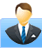Register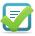Login

IT Strategic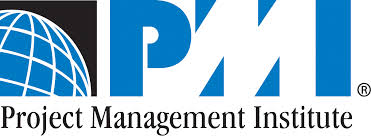PMP Scope Management (210),Integration Management(240),Cost Management(180),Time Management(120),HR Management(210),Risk Management(120),Communication Managment(150),Quality Management(150),Procurement Management(150) Total no of Question:3000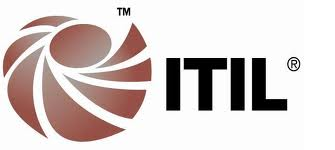ITIL Foundation V3 Coming Soon Total no of Question:300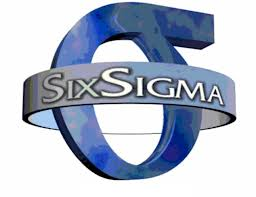Six Sigma Green Belt Define(30),Measure(30),Analyze(30),Improve(30),Control(30) Total no of Question:150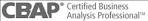BABOK Business Analysis Planning and Monitoring(30),Requirements Management and Communication(30),Enterprise Analysis(30),Elicitation(30),Requirements Analysis(30),Solution Assessment and Validation(30) Total no of Question:180APTITUDE More than 2000 APTITUDE practice questions in various areas of Number Theory, Comparison, Measurement, Skip Counting, Place Value, Geometry, Addition, Subtraction, Multiplication, Division, Decimal, Data Chart Graph, Time, Probability, Estimation and Rounding, Equation, Fraction, Algebra and Function. Total no of Question:2000
Kids Mathematics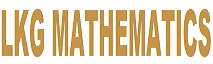Pre - KG/LKG Mathematics More than 500 Mathematics practice questions in various areas of Number Sense, Number Theory, Comparison, Skip Counting, Position, Classify, Measurement, Geometry. Total no of Question:500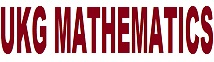Kindergarten/UKG Mathematics More than 1000 Mathematics practice questions in various areas of Number Sense, Number Theory, Comparison, Skip Counting, Number Line, Position, Classify, Measurement, Geometry, Place Value, Addition, Subtraction, Time. Total no of Question:1000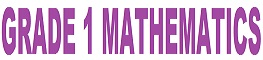Grade 1 Mathematics More than 1500 Mathematics practice questions in various areas of Number Sense, Number Theory, Comparison, Skip Counting, Number Line, Position, Classify, Measurement, Geometry, Place Value, Addition, Subtraction, Time. Total no of Question:1500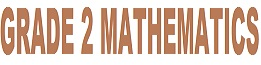Grade 2 Mathematics More than 2000 Mathematics practice questions in various areas of Number Theory, Comparison, Measurement, Skip Counting, Place Value, Geometry, Addition, Subtraction, Multiplication, Division, Data Chart Graph, Time, Estimation and Rounding, Equation. Total no of Question:1500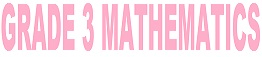Grade 3 Mathematics More than 2000 Mathematics practice questions in various areas of Number Theory, Comparison, Measurement, Skip Counting, Place Value, Geometry, Addition, Subtraction, Multiplication, Division, Decimal, Data Chart Graph, Time, Probability, Estimation and Rounding, Equation, Fraction, Algebra. Total no of Question:1500Grade 4 Mathematics More than 2000 Mathematics practice questions in various areas of Number Theory, Comparison, Measurement, Skip Counting, Place Value, Geometry, Addition, Subtraction, Multiplication, Division, Decimal, Data Chart Graph, Time, Probability, Estimation and Rounding, Equation, Fraction, Algebra and Function. Total no of Question:1500Grade 5 Mathematics More than 2000 Mathematics practice questions in various areas of Number Theory, Comparison, Measurement, Skip Counting, Place Value, Geometry, Addition, Subtraction, Multiplication, Division, Decimal, Data Chart Graph, Time, Probability, Estimation and Rounding, Equation, Fraction, Algebra, Function, Ration, and Percentage. Total no of Question:1500
Kids EnglishPre - KG/LKG English Student will understand: Letter wise picture, Mapping of capital and small letter. Practice around 200 questions Total no of Question:200Kindergarten/UKG English Student will understand: Letter wise picture, Mapping of capital and small letter. Practice around 200 questions Total no of Question:200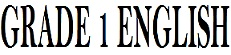Grade 1 English Student will understand and improve: Writing skill, Grammer, Punctuation Rules, Vocabulary, Modal, Reading Comprhension, Word Definition and Sentence completion etc.... Practice around 1000 questions Total no of Question:1000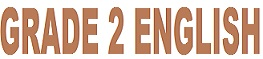Grade 2 English Student will understand and improve: Writing skill, Grammer, Punctuation Rules, Vocabulary, Modal, Reading Comprhension, Word Definition and Sentence completion etc.... Practice around 1000 questions Total no of Question:1000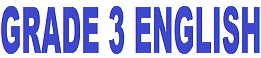Grade 3 English Student will understand and improve: Writing skill, Grammer, Punctuation Rules, Vocabulary, Modal, Reading Comprhension, Word Definition and Sentence completion etc.... Practice around 1000 questions Total no of Question:1000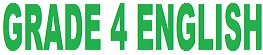Grade 4 English Student will understand and improve: Writing skill, Grammer, Punctuation Rules, Vocabulary, Modal, Reading Comprhension, Word Definition and Sentence completion etc.... Practice around 1000 questions Total no of Question:1000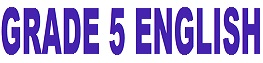Grade 5 English Student will understand and improve: Writing skill, Grammer, Punctuation Rules, Vocabulary, Modal, Reading Comprhension, Word Definition and Sentence completion etc.... Practice around 1000 questions Total no of Question:1000
K-12 competitive Test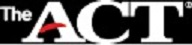ACT Examination Practice 10 set of practical ACT Mathematics examinations. Covered all the topics Pre-Algebra, Elementary Algebra, Intermediate Algebra, Coordinate Geometry, Plane Geometry, Trigonometry. You can appear the examination anytime anywhere. You can appear the examination any number of times also. Total no of Question:600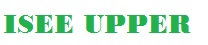ISEE Upper Level Practice 15 set of practical ISEE Upper Level Mathematics examinations. You can appear the examination anytime anywhere. You can appear the examination any number of times also. Total no of Question:705GED Practice 15 set of practical GED Mathematics examinations. You can appear the examination anytime anywhere. You can appear the examination any number of times also. Total no of Question:15 Sets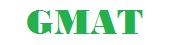GMAT Practice 15 set of practical GMAT Mathematics examinations. You can appear the examination anytime anywhere. You can appear the examination any number of times also. Total no of Question:15 Sets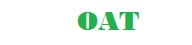OAT Practice 15 set of practical OAT Mathematics examinations. You can appear the examination anytime anywhere. You can appear the examination any number of times also. Total no of Question:15 Sets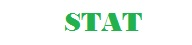STAT Practice 15 set of practical STAT Mathematics examinations. You can appear the examination anytime anywhere. You can appear the examination any number of times also. Total no of Question:15 Sets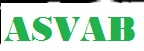ASVAB Practice 15 set of practical ASVAB Mathematics examinations. You can appear the examination anytime anywhere. You can appear the examination any number of times also. Total no of Question:15 Sets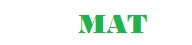MAT Practice 15 set of practical MAT Mathematics examinations. You can appear the examination anytime anywhere. You can appear the examination any number of times also. Total no of Question:15 Sets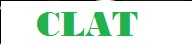CLAT Practice 15 set of practical CLAT Mathematics examinations. You can appear the examination anytime anywhere. You can appear the examination any number of times also. Total no of Question:15 Sets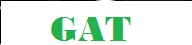GAT Practice 15 set of practical GAT Mathematics examinations. You can appear the examination anytime anywhere. You can appear the examination any number of times also. Total no of Question:15 SetsPSATNMSQT Practice 15 set of practical PSATNMSQT Mathematics examinations. You can appear the examination anytime anywhere. You can appear the examination any number of times also. Total no of Question:15 Sets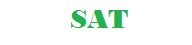SAT Practice 15 set of practical SAT Mathematics examinations. You can appear the examination anytime anywhere. You can appear the examination any number of times also. Total no of Question:15 Sets
Manners and Etiquette for Children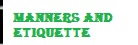Manners and Etiquette for Children General Manners and Etiquette for Children, Child Safety measures in School Bus, Child Safety measures in Swimming pool, Child Safety measures for school picnic, Child Safety measures in riding Bike, Child Safety measures on Road, Child Safety measures in Home, Manners and Etiquette in Prayer Room, Manners and Etiquette at Restaurant, Manners and Etiquette at Clinic / Hospital, Manners and Etiquette at Playground, Manners and Etiquette in School, Manners and Etiquette in Classroom, Table Manner, Home Manner, Be a Kind classmate, Inspiring Quotes for Kids, Morals of various stories and lessons, Lessons Learn from Story, Activity to Physically fit, Manner with Elders, Punctuality, Gartitude Total no of Question:600